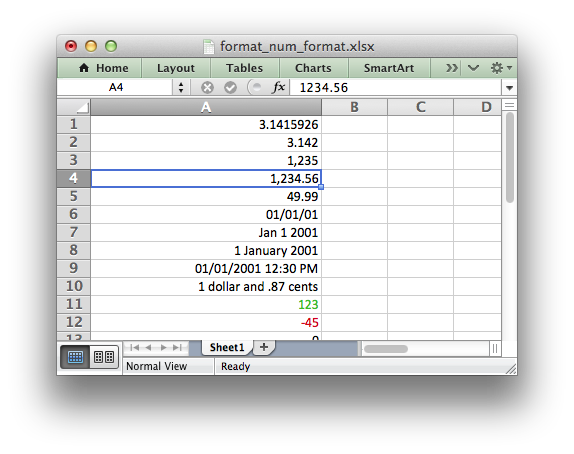libxlsxwriter
format_num_format.c

Example of writing some data with numeric formatting to a simple Excel file using libxlsxwriter./*
* Example of writing some data with numeric formatting to a simple Excel file
* using libxlsxwriter.
*
* Copyright 2014-2021, John McNamara, jmcnamara@cpan.org
*
*/
#include "xlsxwriter.h"
int main() {
/* Create a new workbook and add a worksheet. */
lxw_workbook *workbook = workbook_new("format_num_format.xlsx");
/* Widen the first column to make the text clearer. */
worksheet_set_column(worksheet, 0, 0, 30, NULL);
/* Set some example number formats. */
format_set_num_format(format01, "0.000");
format_set_num_format(format02, "#,##0");
format_set_num_format(format03, "#,##0.00");
format_set_num_format(format04, "0.00");
format_set_num_format(format05, "mm/dd/yy");
format_set_num_format(format06, "mmm d yyyy");
format_set_num_format(format07, "d mmmm yyyy");
format_set_num_format(format08, "dd/mm/yyyy hh:mm AM/PM");
format_set_num_format(format09, "0 \"dollar and\" .00 \"cents\"");
/* Write data using the formats. */
worksheet_write_number(worksheet, 0, 0, 3.1415926, NULL); // 3.1415926
worksheet_write_number(worksheet, 1, 0, 3.1415926, format01); // 3.142
worksheet_write_number(worksheet, 2, 0, 1234.56, format02); // 1,235
worksheet_write_number(worksheet, 3, 0, 1234.56, format03); // 1,234.56
worksheet_write_number(worksheet, 4, 0, 49.99, format04); // 49.99
worksheet_write_number(worksheet, 5, 0, 36892.521, format05); // 01/01/01
worksheet_write_number(worksheet, 6, 0, 36892.521, format06); // Jan 1 2001
worksheet_write_number(worksheet, 7, 0, 36892.521, format07); // 1 January 2001
worksheet_write_number(worksheet, 8, 0, 36892.521, format08); // 01/01/2001 12:30 AM
worksheet_write_number(worksheet, 9, 0, 1.87, format09); // 1 dollar and .87 cents
/* Show limited conditional number formats. */
format_set_num_format(format10, "[Green]General;[Red]-General;General");
worksheet_write_number(worksheet, 10, 0, 123, format10); // > 0 Green
worksheet_write_number(worksheet, 11, 0, -45, format10); // < 0 Red
worksheet_write_number(worksheet, 12, 0, 0, format10); // = 0 Default color
/* Format a Zip code. */
format_set_num_format(format11, "00000");
worksheet_write_number(worksheet, 13, 0, 1209, format11);
/* Close the workbook, save the file and free any memory. */
return workbook_close(workbook);
}
workbook_close
lxw_error workbook_close(lxw_workbook *workbook)
Close the Workbook object and write the XLSX file.
workbook_new
lxw_workbook * workbook_new(const char *filename)
Create a new workbook object.
format_set_num_format
void format_set_num_format(lxw_format *format, const char *num_format)
Set the number format for a cell.
lxw_worksheet
Struct to represent an Excel worksheet.
Definition: worksheet.h:2107
lxw_format
Struct to represent the formatting properties of an Excel format.
Definition: format.h:358
lxw_workbook
Struct to represent an Excel workbook.
Definition: workbook.h:292
worksheet_write_number
lxw_error worksheet_write_number(lxw_worksheet *worksheet, lxw_row_t row, lxw_col_t col, double number, lxw_format *format)
Write a number to a worksheet cell.
worksheet_set_column
lxw_error worksheet_set_column(lxw_worksheet *worksheet, lxw_col_t first_col, lxw_col_t last_col, double width, lxw_format *format)
Set the properties for one or more columns of cells.